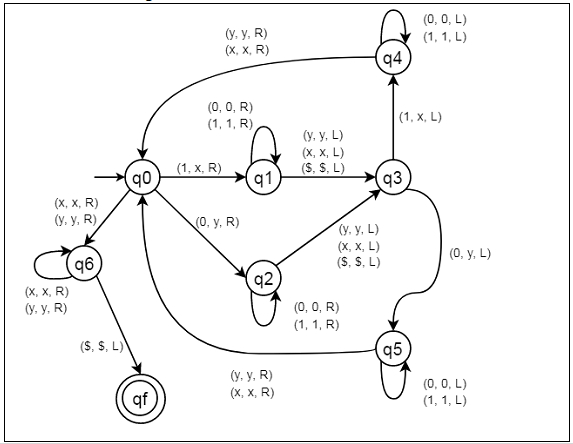# Construct a Turing Machine for language L = {wwr | w ã {0, 1}}

Here we will see how to make a Turing machine for language L = {WWr |W belongs to {0, 1}}. So this represents a kind of language where we will use only two characters 0s and 1s. The w is a string and wr is reverse of it. So if w = 10110, then wr will be 01101. So the Turing machine will accept the string z = 1011001101.

To solve this, we will use this approach. First check the first symbol, if it’s 0 then replace it using y and if that is 1, then replace using x. Then go to the end of string. So last symbol is same as the first one. We replace it also by x or y depending on it. After that again come back to the position next to the symbol replace from the starting and repeat the same process that is mentioned above. We have to keep in mind that since wr is reverse of w of both of them will have equal number of symbols. Every time replace a nth symbol from beginning of string, replace a corresponding nth symbol from the end.

## State Transition Diagram